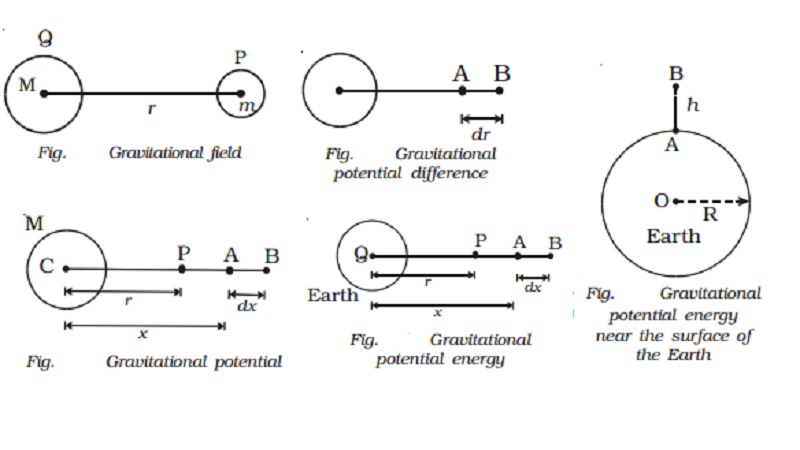Home | | Physics | | Physics | Gravitational field: potential difference and potential energy

# Gravitational field: potential difference and potential energyGravitational field: Gravitational potential difference, Gravitational potential, Expression for gravitational potential at a point, Gravitational potential energy, Gravitational potential energy near the surface of the Earth

Gravitational field

Two masses separated by a distance exert gravitational forces on one another. This is called action at-a-distance. They interact even though they are not in contact. This interaction can also be explained with the field concept. A particle or a body placed at a point modifies a space around it which is called gravitational field. When another particle is brought in this field, it experiences gravitational force of attraction.

The gravitational field is defined as the space around a mass in which it can exert gravitational force on other mas.

Gravitational field intensity

Gravitational field intensity or strength at a point is defined as the force experienced by a unit mass placed at that point. It is denoted by E. It is a vector quantity. Its unit is N kg-1.

Consider a body of mass M placed at a point Q and another body of mass m placed at P at a distance r from Q.The mass M develops a field E at P and this field exerts a force

F = mE.

The gravitational force of attraction between the masses m and M is F = GMm/r2

The gravitational field intensity at P is E = F/m

E = GM/r2

Gravitational field intensity is the measure of gravitational field.

Gravitational potential difference

Gravitational potential difference between two points is defined as the amount of work done in moving unit mass from one point to another point against the gravitational force of attraction.Consider two points A and B separated by a distance dr in the gravitational field.

The work done in moving unit mass from A to B is dv = WA → B

Gravitational potential difference dv = − E dr

Here negative sign indicates that work is done against the gravitational field.

Gravitational potential

Gravitational potential at a point is defined as the amount of work done in moving unit mass from the point to infinity against the gravitational field. It is a scalar quantity. Its unit is N m kg-1.

Expression for gravitational potential at a point

Consider a body of mass M at the point C. Let P be a point at a distance r from C. To calculate the gravitational potential at P consider two points A and B. The point A, where the unit mass is placed is at a distance x from C.The gravitational field at A is E = GM/x2

The work done in moving the unit mass from A to B through a small distance dx is dw = dv = −E.dx

dv = − ( GM/x2 ) dx

The work done in moving the unit mass from the point P to infinity is

∫dv = -  ∫(GM/x2) dx

v= - GM/r

The gravitational potential is negative, since the work is done against the field. (i.e) the gravitational force is always attractive.

Gravitational potential energy

Consider a body of mass m placed at P at a distance r from the centre of the Earth. Let the mass of the Earth be M.

When the mass m is at A at a distance x from Q, the gravitational force of attraction on it due to mass M is given by F = GMm / x2

The work done in moving the mass m through a small distance dx from A to B along the line joining the

two centres of masses m and M is dw = -F.dx.

dw = - GMm / x2 dxThe gravitational potential energy of a mass m at a distance r from another mass M is defined as the amount of work done in moving the mass m from a distance r to infinity.

The total work done in moving the mass m from a distance r to infinity is

*U = GMm/r

Gravitational potential energy is zero at infinity and decreases as the distance decreases. This is due to the fact that the gravitational force exerted on the body by the Earth is attractive. Hence the gravitational potential energy U is negative.

Gravitational potential energy near the surface of the Earth

Let the mass of the Earth be M and its radius be R. Consider a point A on the surface of the Earth and another point B at a height h above the surface of the Earth. The work done in moving the mass m from A to B is U = UB − UAU = GMmh / R(R+h)

If the body is near the surface of the Earth, h is very small when compared with R. Hence (R+h) could be taken as R.

U = GMmh / R2

U = mgh

Study Material, Lecturing Notes, Assignment, Reference, Wiki description explanation, brief detail

Related Topics